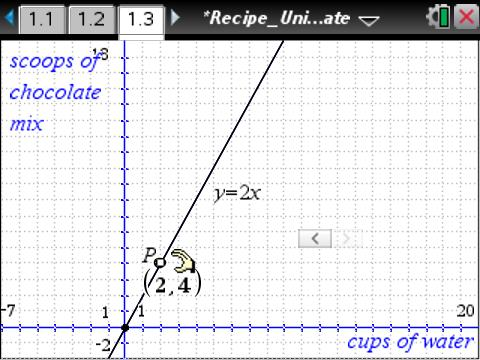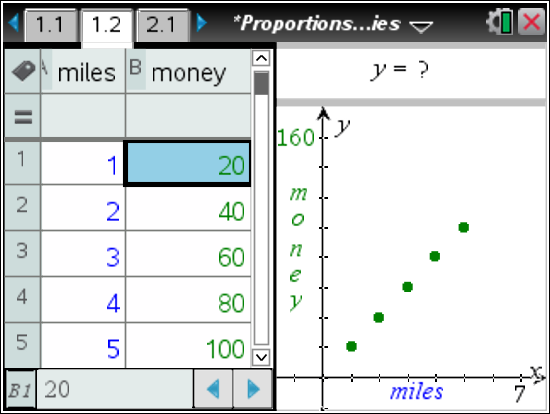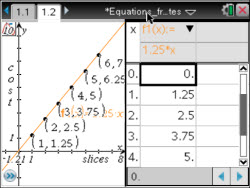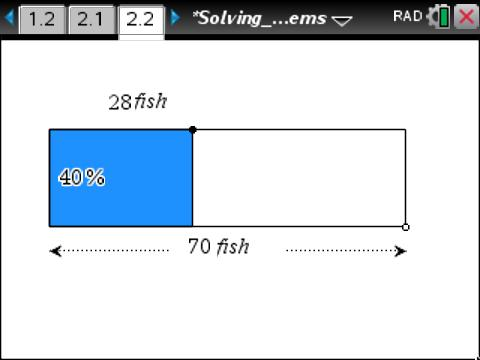### How Does a Spring Scale Work?

In this lesson, teachers will use a spring to help students learn that the constant of proportionality between two proportional quantities is the unit rate of change.
•TI-Nspire™ CX/CX II
•TI-Nspire™ CX CAS/CX II CAS
• TI-Nspire™ Navigator™### Recipe: Unit Rate

Students look at unit rate in a real-world context. They will use ratios to create points, plot them, and determine the mathematical relationship for the plotted points. Then, they will predict other points based on the relationship determined.
•TI-Nspire™ CX/CX II
•TI-Nspire™ CX CAS/CX II CAS
• TI-Nspire™ Navigator™
• TI-Nspire™
• TI-Nspire™ CAS### Proportionality in Tables, Graphs, and Equations

Students will recognize and identify the characteristics of proportional and non-proportional relationships by analyzing a table, equation, and graph.
•TI-Nspire™ CX/CX II
•TI-Nspire™ CX CAS/CX II CAS
• TI-Nspire™ Navigator™
• TI-Nspire™
• TI-Nspire™ CAS### Proportions in Stories

This lesson involves inputting data into a table, observing the data on coordinate plane, and inputting an equation that corresponds with the table of values.
•TI-Nspire™ CX/CX II
•TI-Nspire™ CX CAS/CX II CAS
• TI-Nspire™ Navigator™
• TI-Nspire™
• TI-Nspire™ CAS### Equations from Unit Rates

This lesson involves finding a linear equation and confirming the equation represents a proportional relationship with numeric values in ordered pairs or in functions tables.
•TI-Nspire™ CX/CX II
•TI-Nspire™ CX CAS/CX II CAS
• TI-Nspire™ Navigator™
• TI-Nspire™
• TI-Nspire™ CAS### Solving Percent Problems

This lesson involves solving word problems dealing with percents by using visual and numerical representations of percents.
•TI-Nspire™ CX/CX II
•TI-Nspire™ CX CAS/CX II CAS